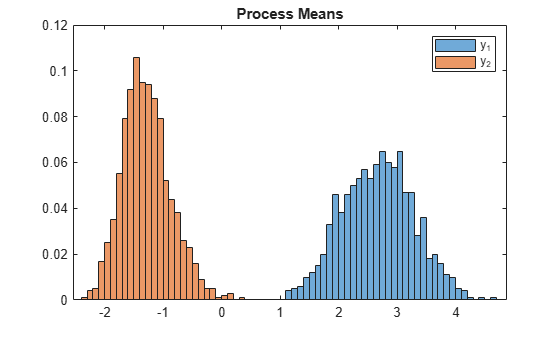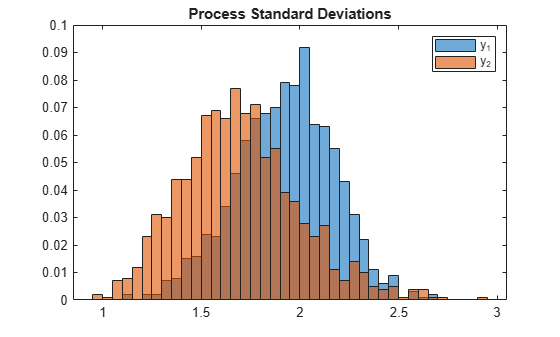# Monte Carlo Simulation of Markov-Switching Dynamic Regression Model Response Variables

This example shows how to characterize the distribution of a multivariate response series, modeled by a Markov-switching dynamic regression model, by summarizing the draws of a Monte Carlo simulation.

Consider the response processes ${\mathit{y}}_{1\mathit{t}}$ and ${\mathit{y}}_{2\mathit{t}}$ that switch between three states, governed by the latent process ${\mathit{s}}_{\mathit{t}}$ with this observed transition matrix:

`$P=\left[\begin{array}{ccc}10& 1& 1\\ 1& 10& 1\\ 1& 1& 10\end{array}\right].$`

Suppose that ${y}_{t}={\left[\begin{array}{cc}{y}_{1t}& {y}_{2t}\end{array}\right]}^{\prime }$ is a VARX model in each state:

`${y}_{t}=\left\{\begin{array}{ll}\left[\begin{array}{c}1\\ -1\end{array}\right]+{\epsilon }_{1t}& ;{s}_{t}=1\\ \left[\begin{array}{c}2\\ -2\end{array}\right]+\left[\begin{array}{cc}0.5& 0.1\\ 0.5& 0.5\end{array}\right]{y}_{t-1}+{\epsilon }_{2t}& ;{s}_{t}=2\\ \left[\begin{array}{c}3\\ -3\end{array}\right]+\left[\begin{array}{cc}0.25& 0\\ 0& 0\end{array}\right]{y}_{t-1}+\left[\begin{array}{cc}0& 0\\ 0.25& 0\end{array}\right]{y}_{t-2}+{\epsilon }_{3t}& ;{s}_{t}=3,\end{array}$`

where, for j = 1,2,3, ${\epsilon }_{\mathrm{jt}}$ is Gaussian with mean 0 and covariance matrix

`${\Sigma }_{j}=j\left[\begin{array}{cc}1& -0.1\\ -0.1& 1\end{array}\right].$`

### Create Fully Specified Model

Create the Markov-switching dynamic regression model that describes ${\mathit{y}}_{\mathit{t}}$ and ${\mathit{s}}_{\mathit{t}}$.

```% Switching mechanism P = [10 1 1; 1 10 1; 1 1 10]; mc = dtmc(P); % VAR submodels C1 = [1;-1]; C2 = [2;-2]; C3 = [3;-3]; AR1 = {}; AR2 = {[0.5 0.1; 0.5 0.5]}; AR3 = {[0.25 0; 0 0] [0 0; 0.25 0]}; Beta1 = [1; -1]; Beta2 = [2 2;-2 -2]; Beta3 = [3 3 3;-3 -3 -3]; Sigma1 = [1 -0.1; -0.1 1]; Sigma2 = [2 -0.2; -0.2 2]; Sigma3 = [3 -0.3; -0.3 3]; mdl1 = varm(Constant=C1,AR=AR1,Beta=Beta1,Covariance=Sigma1); mdl2 = varm(Constant=C2,AR=AR2,Beta=Beta2,Covariance=Sigma2); mdl3 = varm(Constant=C3,AR=AR3,Beta=Beta3,Covariance=Sigma3); Mdl = msVAR(mc,[mdl1; mdl2; mdl3]);```

`Mdl` is a fully specified `msVAR` object.

### Simulate Multiple Paths

Simulate 1000 separate, independent paths of responses from the model. Specify a 50-period simulation horizon. Start all simulations in the first state (that is, the state of the system at time 0 is state 1), by specifying a distribution so that state 1 has all mass.

```rng("default") % For reproducibility s0 = [1 0 0]; Y = simulate(Mdl,50,NumPaths=1000,S0=s0);```

`Y` is a 50-by-2-by-1000 array of simulated responses. Each page is a simulated path from `Mdl`.

### Summarize Monte Carlo Draws

The processes are stationary. Therefore, for each path, obtain the typical value of each variable by computing the sample mean.

`mus = mean(Y,1);`

`mus` is a 1-by-2-by-1000 array. Each page is the sample mean vector of the simulated path.

Plot the Monte Carlo probability distribution of the response means.

```figure histogram(mus(1,1,:),Normalization="probability",BinWidth=0.1); hold on histogram(mus(1,2,:),Normalization="probability",BinWidth=0.1); legend("y_1","y_2") title("Process Means") hold off```For each path, obtain the variability of each variable by computing the sample standard deviation. Plot the Monte Carlo probability distribution of the response standard deviations.

```sigmas = std(Y,0,1); figure histogram(sigmas(1,1,:),Normalization="probability",BinWidth=0.05) hold on histogram(sigmas(1,2,:),Normalization="probability",BinWidth=0.05) legend("y_1","y_2") title("Process Standard Deviations") hold off```